# Time sequence for a Gaussian beam

The time sequence for a collimated Gaussian-field beam laser pulse entering a nonscattering absorbing medium at an air/medium interface.

Absorption coefficient μa = 50 cm-1 = 5000 m-1.
Irradiance E = 0.0200 [J/cm2] = 200 [J/m2].
Energy deposition W = μaE = 1 [J/cm3] = 106 [J/m3].
Peak pressure = ΓW =(0.12)(106 [J/m3]) = 1.2x105 [J/m3] = 1.2x105 [Pa].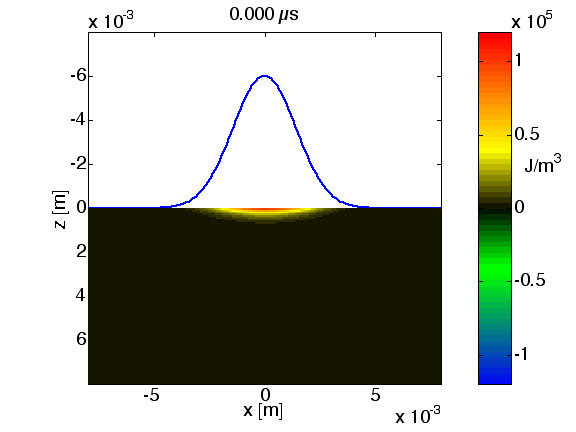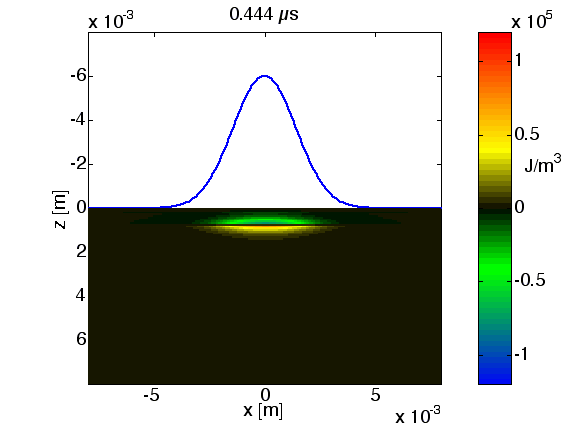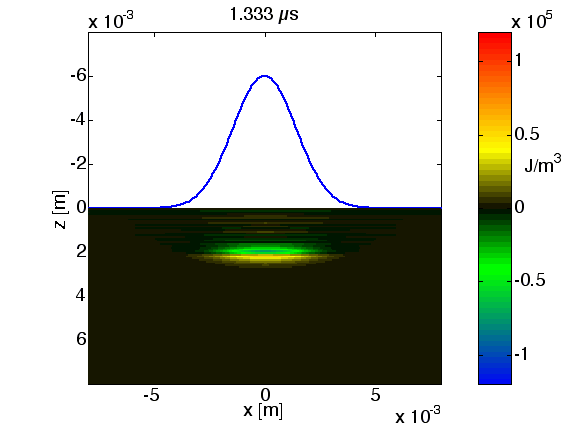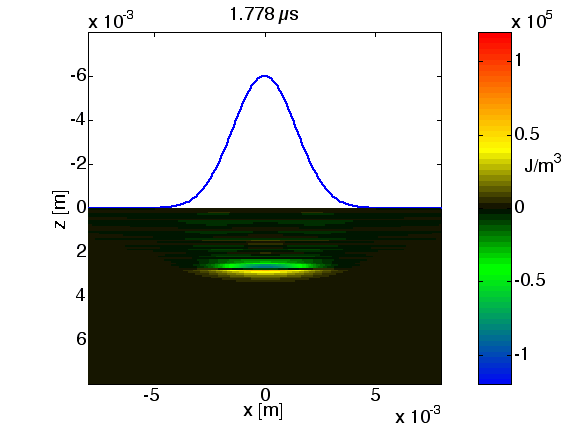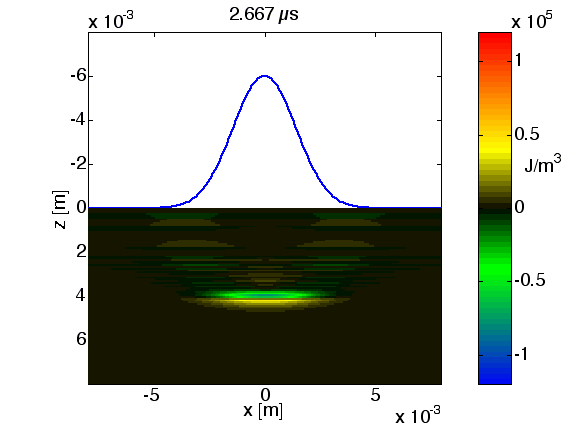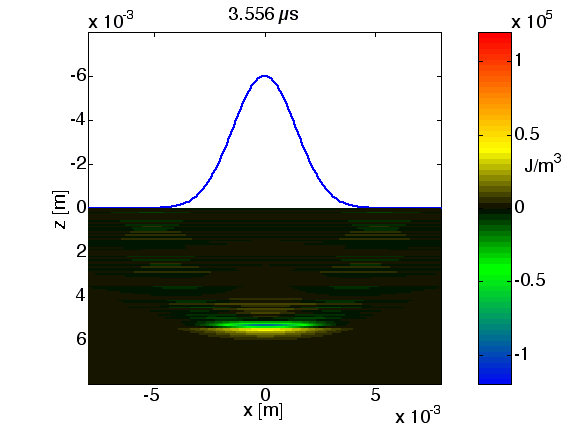Again, the key features are the leading positive compressive pressure wave (red) and negative tensile pressure wave (blue). However, the Gaussian shape does not have the sharp edges of the flat-field beam. Consequently, the pattern of pressure is significantly different than the pressure pattern induced by a flat-field beam.

This early computation still suffers from some computational noise in the low pressure regions, so do not attach too much significance to pressures fluctuating close to zero (slight green or yellow). Work in progress :-)